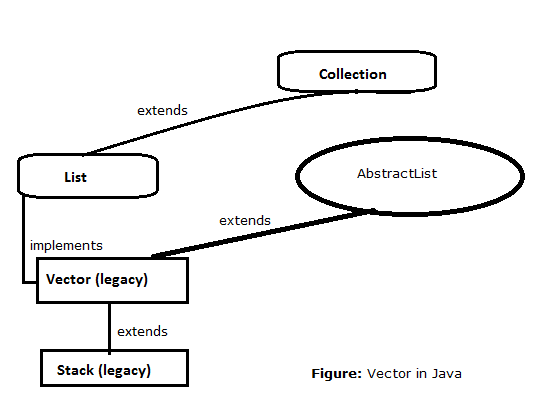Vector class

In this article, we will discuss Vector class – one of the List implemented class in detail

• Vector is a legacy class
• introduced in Java 1.0 version
• all its method are synchronized i.e.; thread-safe for any operation

Vector:

• Vector is implementation class of List interface (i.e.; Vector implements List)
• Vector uses resizable array or grow-able array to store element/objects
• Duplicate element/objects are allowed to be inserted
• Insertion order is maintained
• Allows NULL insertion (no limit, any number of NULL insertion is allowed)
• Without generics, Vector allows to insert any type of objects;
• with generics, it is type-bounded (except, if we take Object as type within angle brackets)
• Elements retrieval is faster as Vector works on zero-based index
• Manipulation (i.e.; addition/deletion of element from middle of Vector) is very slow, as it requires lot of shifting work internally
• Note: this is exactly same as that of ArrayList except all methods of Vector class is synchronized. Hence, it is thread-safe
• Present in java.util package and extends java.util.AbstractList implements java.util.List interface
• Also, implements java.util.RandomAccess, java.lang.Cloneable, java.io.Serializable marker interfaces which provides special ability to Vector (provided by JVM at run time) like
• java.util.Random Access: to access any random element/objects with same speed
• java.lang.Cloneable: to create a duplicate object or to clone an object
• java.io.Serializable: to transfer objects across networkSource: Team BenchResources.Net

Vector constructors:

Vector v = new Vector();

• creates an empty Vector object of size 10
• when maximum size of the Vector is reached, then new Vector of bigger size is created using below formula
Capacity = New Vector size = 2 * current size (double in size)
• old Vector item values will be copied into new Vector

Vector v = new Vector(int initialCapacity);

• creates an empty Vector object of specified size (or initial capacity)
• when maximum size is reached, above formula will be applied and new ArryaList will be created

Vector v = new Vector(int initialCapacity, int incrementalCapacity);

• creates an empty Vector object of specified size (or initial capacity)
• when maximum size is reached, new vector is created with a size of (old vector size + increment capacity value)

Vector v = new Vector(Collection c);

• creates an equivalent Vector object for the specified collection
• it is basically used for inter-conversion between collection objects

Vector methods:

• Vector specific methods from Java 1.0 version
 Vector method Description void addElement(Object obj); add/inserts specified element/object at the end of the invoking vector boolean removeElement(Object obj); removes first occurrence of specified element/object from the invoking vector void removeElementAt(int index); removes element/object from the invoking vector at the specified index void removeAllElements(); removes all element/objects from the invoking vector after removing all element/objects, size of vector will become zero Object elementAt(int index); returns element/object from the invoking vector at the specified index Object firstElement(); returns first element/object from the invoking vector Object lastElement(); returns last element/object from the invoking vector int size(); returns number of elements present in the invoking vector (at the time of invoking) int capacity(); returns current capacity of the invoking vector Enumeration elements(); returns Enumeration of the elements in the invoking vector

Vector examples:

```package in.bench.resources.java.collection;

import java.util.Vector;

public static void main(String[] args) {

// creating Vector object of type String
Vector<String> vec = new Vector<String>();

// adding elements to Vector object

System.out.println("Iterating Vector values\n");

// Iterating using enhanced for-loop
for(String str : vec){
System.out.println(str);
}

// removing element at 4th index
vec.removeElement(4);

// to print all values of Vector
System.out.println("\n\nVector values after removal "
+ "at 4th index postion \n" + vec);
}
}
```

Output:

```Iterating Vector values

Sundar Pichai
Shantanu Narayen
Sundar Pichai
Francisco D’Souza

Vector values after removal at 4th index postion